Similar Figures (Sides)
Similar Figures (Angles)
Transformations
Angles of Triangles
Dilations & Scale Factor
100

The figures are similar. Find x.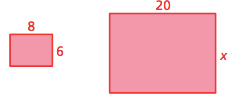15

100

Are the triangles similar?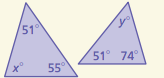Yes

100

The vertices of a triangle are A(3,5), B(-2,4), and C(-2,-5).

Apply the transformation (x,y) --> (x-1,y+4) to the vertices of the original triangle and give the coordinates of each vertex of the image. Then name the type of transformation (translation, reflection, rotation, dilation).

A'(2,9)

B'(-3,8)

C'(-3,-1)

Translation

100

Find the measures of the interior angles40, 65, 75

100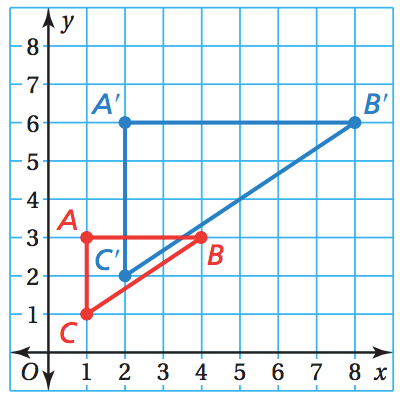The blue figure is a dilation of the red figure. Identify the type of dilation and find the scale factor.

Enlargement, 2

200

The figures are similar. Find x.14

200

Which one doesn't belong?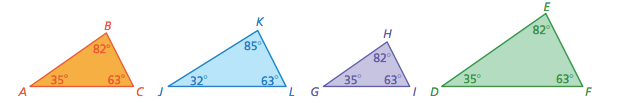Triangle JKL

200

The vertices of a triangle are A(3,5), B(-2,4), and C(-2,-5).

Apply the transformation (x,y) --> (2x,2y) to the vertices of the original triangle and give the coordinates of each vertex of the image. Then name the type of transformation (translation, reflection, rotation, dilation).

A'(6,10)

B'(-4,8)

C'(-4,-10)

Dilation

200

Find the measure of the exterior angle.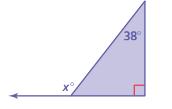128

200

Which figure is not a dilation of the blue figure?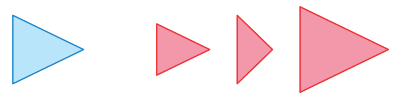Middle

300

The figures are similar. Find x.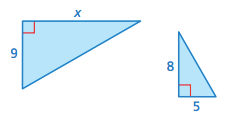14.4

300

Triangle EFG and some of its measurements are shown. Triangle ABC is similar to triangle EFG. What is the measure of angle B?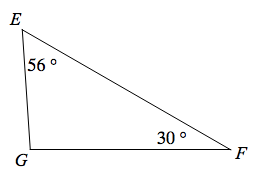30

300

The vertices of a triangle are A(3,5), B(-2,4), and C(-2,-5).

Apply the transformation (x,y) --> (x,-y) to the vertices of the original triangle and give the coordinates of each vertex of the image. Then name the type of transformation (translation, reflection, rotation, dilation).

A'(3,-5)

B'(-2,-4)

C'(-2,5)

Reflection

300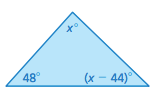Find the measures of the interior angles.

44, 48, 88
300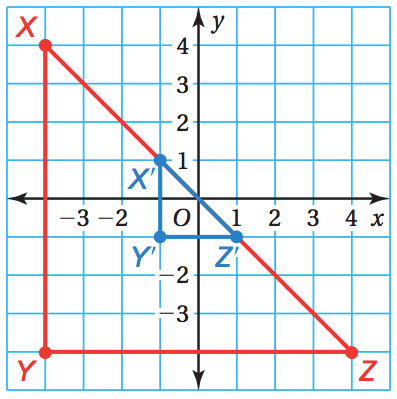The blue figure is a dilation of the red figure. Identify the type of dilation and find the scale factor.

Reduction, 1/4

400

A student’s rectangular desk is 30 inches long and 18 inches wide. The teacher’s desk is similar to the student’s desk and has a length of 50 inches. What is the width of the teacher’s desk?

30in

400

Triangle ABC is similar to triangles DEF. What is the measure of angle F?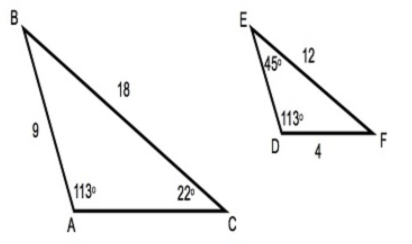22

400

The vertices of a triangle are A(3,5), B(-2,4), and C(-2,-5).

Apply the transformation (x,y) --> (-x,y) to the vertices of the original triangle and give the coordinates of each vertex of the image. Then name the type of transformation (translation, reflection, rotation, dilation).

A'(-3,5)

B'(2,4)

C'(2,-5)

Reflection

400

Find the measure of the exterior angle.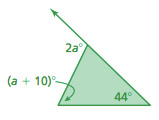108

400

A triangle is dilated using a scale factor of 8. The image is then dilated using a scale factor of 1/2. What scale factor could you use to dilate the original triangle to get the final image?

4

500

A Mexican flag is 63 inches long and 36 inches wide. Is the drawing similar to the Mexican flag?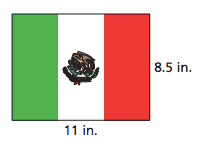no

500

Triangle XYZ is similar to triangle LMN. If the measure of angle Z is 35 degrees, what is the measure of angle N?

35

500

The vertices of a triangle are A(3,5), B(-2,4), and C(-2,-5).

Apply the transformation (x,y) --> (y,-x) to the vertices of the original triangle and give the coordinates of each vertex of the image. Then name the type of transformation (translation, reflection, rotation, dilation).

A'(5,-3)

B'(4,2)

C'(-5,2)

Rotation

500

The triangle with lines through it designates a no parking zone. What is the value of x?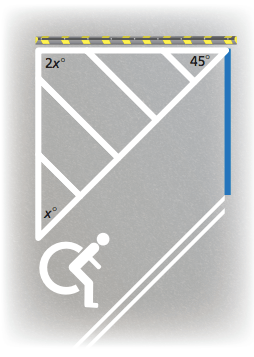45

500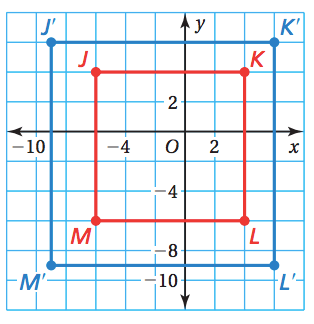The blue figure is a dilation of the red figure. Identify the type of dilation and find the scale factor.

Enlargement, 3/2

Click to zoom